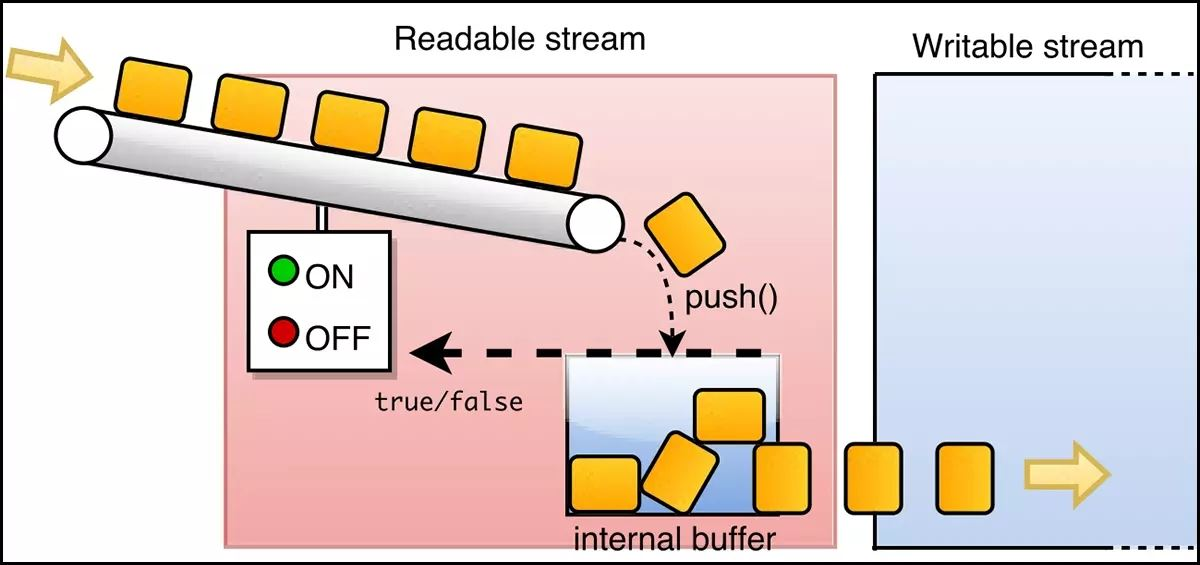# NodeJS实现自定义流的方法stream 模块介绍

``````
let { Readable } = require("stream");

// 创建自定义可读流的类
constructor() {
super();
this.index = 0;
}

this.index++;
this.push(this.index + "");

if (this.index === 3) {
this.push(null);
}
}
}

``````

2、验证自定义可读流

``````

console.log(data);
});

console.log("读取完成");
});

// <Buffer 31>
// <Buffer 32>
// <Buffer 33>
// 读取完成``````

1、创建自定义可写流的类 MyWrite

``````
let { Writable } = require("stream");
// 创建自定义可写流的类
class MyWrite extends Writable {
// 重写自定义的可写流的 _write 方法
_write(chunk, encoding, callback)) {
callback(); // 将缓存区写入文件
}
}``````

2、验证自定义可写流

``````
let myWrite = new MyWrite();
myWrite.write("hello", "utf8", () => {
console.log("hello ok");
});
myWrite.write("world", "utf8", () => {
console.log("world ok");
});
// hello ok
// world ok``````

1、创建自定义可双工流的类 MyDuplex

``````
let { Duplex } = require("stream");
// 创建自定义双工流的类
class MyDuplex extends Duplex {
this.push("123");
this.push(null);
}
// 重写自定义的双工流的 _write 方法
_write(chunk, encoding, callback)) {
callback();
}
}``````

2、验证自定义双工流

``````
let myDuplex = new MyDuplex();
});
setTimeout(() => {
myDuplex.on("data", data => {
console.log(data, "xxxx");
});
}, 3000);
// <Buffer 31> ----
// <Buffer 32> xxxx
// <Buffer 32> ----
// <Buffer 33> xxxx``````

1、创建自定义可转化流的类 MyTransform

``````
let { Transform } = require('stream');
// 创建自定义转化流的类
class MyTransform extends Transform {
// 重写自定义的转化流的 _transform 方法
_transform(chunk, encoding, callback)) {
console.log(chunck.toString.toUpperCase());
callback();
this.push('123');
}
}``````

2、验证自定义转化流

``````
// demo.js
let myTransForm = new MyTransform();
// 使用标准输入
process.stdin.pipe(myTransForm).pipe(process.stdin);``````

nodejs 实现自定义流 nodejs 自定义流